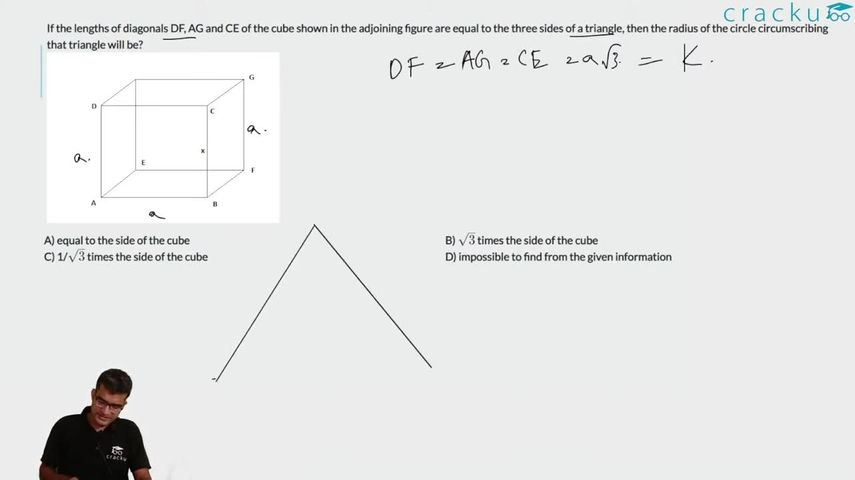Question 25

# If the lengths of diagonals DF, AG and CE of the cube shown in the adjoining figure are equal to the three sides of a triangle, then the radius of the circle circumscribing that triangle will be?Solution

Consider side of the cube as x.

So diagonal will be of length $$\sqrt{3}$$ * x.

Now if diagonals are side of equilateral triangle we get area = 3*$$\sqrt{3}*x^2$$ /4 .

Also in a triangle

4 * Area * R = Product of sides

4* 3*$$\sqrt{3}*x^2$$ /4 * R = .3*$$\sqrt{3}*x^3$$

R = x

### View Video Solution